# From Wavelet Transforms to Convolutional Neural Networks - part 1¶

Autor: Jean Feydy

In this workshop, we're going to classify images using neural networks and nonlinear image transforms. The emphasis will be put on models' architectures: the actual training and visualization code is wrapped into routines such as "evaluate_model" which are located in the "model_utils" file.

The main purpose of these sandbox notebooks is to let you play around with models, "neurons" and convolution filters. As you get to see how these things work under the hood, you should hopefully understand:

• Just how much of a game changer the Autodiff+GPU combo can be: today in Computer Vision (and Natural Language Processing), tomorrow in your own research field - whatever it is.
• How Convolutional Neural Networks can be linked, (at the very least) from an algorithmic perspective, to Wavelet Transforms.
• That the current "Artifical Intelligence" hype around image processing algorithms does not come from scientists. Using momentum-based gradient descent (i.e. letting a heavy ball roll on a hyper-surface of potential) to fine tune the parameters of a Wavelet-like transform can help you to extract the most relevant features in your signal - which is an incredibly useful pre-processing step with tons of industrial applications. But it can not, in any way, model thought. As far as the modelling and understanding of cognitive processes is concerned, we're still very much in the stone age.

Anyway, let's get started. If you haven't done it already, please go through the PyTorch syntax tutorial available here: http://pytorch.org/tutorials/beginner/pytorch_with_examples.html

## Gradient descent in high dimension: the stealth regularization prior¶

In the Deep Learning literature, researchers tend to use (stochastic/momentum-based) gradient descent as their go-to optimization procedure: this algorithm is both simple to implement and efficient in practice. But is it as innocuous/trivial as it seems to be? No, it isn't.

The gradient depends on your underlying metric. As we've seen in the previous workshop sessions, the gradient is fundamentally a metric object. If $f:X\rightarrow Y$ is a differentiable function between two Euclidean/Hilbert spaces, it is defined as the adjoint of the differential seen through the Riesz isomorphism; that is, as the unique application $\partial_x f(x_0) : Y \rightarrow X$ such that

$$\forall\, b \in Y, \forall\, \delta x \in X,~~~ \langle f(x_0+\delta x) \, ,\, b \rangle_Y ~=~ \langle f(x_0) \, ,\, b \rangle_Y ~+~ \langle \delta x \,,\, \partial_x f(x_0).b \rangle_X ~+~ o(\delta x).$$

If $f : \mathbb{R}^N \rightarrow \mathbb{R}$ is a cost function, PyTorch computes its "algorithmic" gradient

$$\partial_x^{L^2} f(x_0):\mathbb{R}\rightarrow \mathbb{R}^N ~\simeq~ ( \partial_{x} f(x_0), \cdots, \partial_{x[N]} f(x_0) )^T$$

is nothing but the $L^2$-gradient associated to the simplistic $L^2$-norm on $X=\mathbb{R}^N$:

$$\forall\, x \in \mathbb{R}^N, ~~ \langle x, x \rangle_X ~=~ \sum_i x[i]^2.$$

More often than not, this choice is a good one; but you should never forget how dependent it is on the way you encoded your data vector $x$: with respect to your real-life problem, the $L^2$ gradient is just as (ir)relevant as the $L^2$ unit ball. Does it make sense? Great. Otherwise, you should be really careful about the bias, the implicit regularization prior you're introducing in your algorithm.

To illustrate this, consider the minimization of a blur-distance function

$$f_{k,y} : x\in \mathbb{R}^N \mapsto \| k\star x - y \|_2^2,$$

where $y \in \mathbb{R}^N$ is a target signal and $k$ is a 1d-convolution kernel. If the Fourier transform of $k$ is never equal to zero, the associated convolution operator is invertible; in this case (say, if $k$ is Gaussian), $f_{k,y}$ is a positive definite quadratic form defined on $\mathbb{R}^N$, whose unique minimum is the well-defined signal

$$x^* ~=~ k^{(-1)} \star y.$$

The strictly convex function $f_{k,y}$ is as simple as it gets, in a finite-dimensional space: therefore, a standard gradient descent (or any similar algorithm) should quickly converge to $x^*$, the unique signal such that $k\star x^* = y$. Let's see how this works in practice.

In :
# PyTorch import
from __future__ import print_function
import torch
import torch.nn as nn
import torch.nn.functional as F
import torch.optim as optim
from torchvision import datasets, transforms, utils
import itertools
import model_utils as MU

# Performance monitoring
from time import process_time
import matplotlib.pyplot as plt
import numpy as np
from scipy.signal import get_window
# Interactive plots "magic line"
%matplotlib nbagg

In :
Resolution = 100 # N = Resolution * 6 + 1

# Generate a sharp, good-looking test 1D signal
t        = np.linspace(0, 6, 6*Resolution + 1)
y        = 0. * t
y[1 < t] = 1.
y[2 < t] = (t[2<t]-3)**2
y[3 < t] = np.sin( 5.5*np.pi*(t[3<t]-3) )**2
y[4 < t] = 5-t[4<t]
y[5 < t] = 0.

y = Variable(torch.from_numpy(y).float(), requires_grad = False )

In :
class BlurDistance(nn.Module) :
"""
Implements a simplistic 1D operator, which applies a gaussian blur
before computing a squared L2 distance to the target y.
"""
def __init__(self, sigma) :
"Defines the parameters of the model."
super(BlurDistance, self).__init__()
# Computes the correct gaussian filter
hsize      = int(2*((6*sigma)//2) + 1)
h          = get_window(('gaussian',sigma), hsize)
h          = h / np.sum(h)   # It will look better
h          = torch.from_numpy(h).float().view(1,1,-1)
# PyTorch isn't really meant to be used with fixed conv filters,
# so the syntax here is not so trivial...
self.conv1 = nn.Conv1d(1, 1, hsize, padding=hsize//2, bias=False)
self.conv1.weight = nn.Parameter( h , requires_grad = False )

def forward(self, x) :
x = self.conv1(x.view(1,1,-1))
return (x, ((x-y)**2).sum() / Resolution)

blur_distance = BlurDistance(.3*Resolution)

In :
# Select the start value for our descent.
# Don't hesitate to play around and try other values!
x = Variable(torch.zeros(y.size()).float(), requires_grad = True )

In :
# Choose the optimizer you like: it won't make much difference
optimizer = optim.SGD( [x], lr = 1., momentum=.5)

In :
loss_descent = []
xs_blurred   = []
for it in range(30001):
(x_blurred, loss) = blur_distance(x)
loss.backward()        # Backpropagation through the model
optimizer.step()       # As gradients have been accumulated, we can make a step
if it%2500 == 0 :
print('It : {:>5}'.format(it), ', Loss = ', loss.data.numpy())
xs_blurred.append(x_blurred) # Store x_blurred, which should converge to y
loss_descent.append(loss.data.cpu().numpy())

It :     0 , Loss =  1.90338
It :  2500 , Loss =  0.18382
It :  5000 , Loss =  0.180069
It :  7500 , Loss =  0.178036
It : 10000 , Loss =  0.176662
It : 12500 , Loss =  0.175666
It : 15000 , Loss =  0.174912
It : 17500 , Loss =  0.17432
It : 20000 , Loss =  0.173841
It : 22500 , Loss =  0.173441
It : 25000 , Loss =  0.173098
It : 27500 , Loss =  0.172798
It : 30000 , Loss =  0.17253

In :
fig = plt.figure()
plt.plot(np.array(loss_descent)[::100])
plt.tight_layout() ; fig.canvas.draw()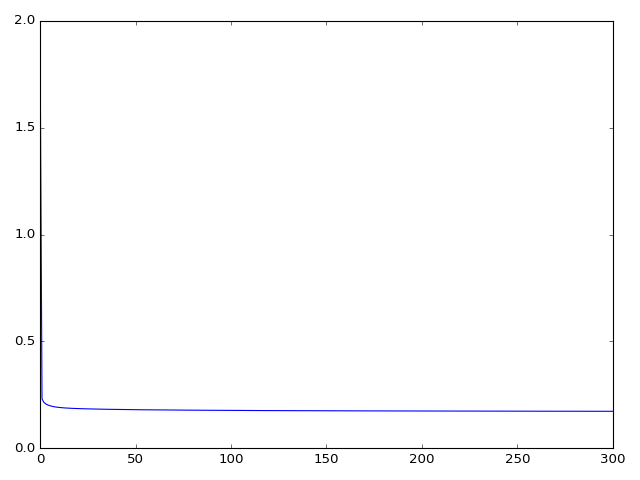After a sharp decline, the algorithm seems to get stuck and converge... towards a value which is not that of the unique critical point of our function, $f_{k,y}(x^*) = 0$. To understand what happened, let's see how $k\star x$ evolved across the iterations.

In :
fig = plt.figure() # (figsize=(5,10))
for xb in xs_blurred :
plt.plot(t,xb.data.cpu().view(-1).numpy())
plt.plot(t, y.data.cpu().view(-1).numpy())
plt.axis('equal') ; plt.tight_layout() ; fig.canvas.draw()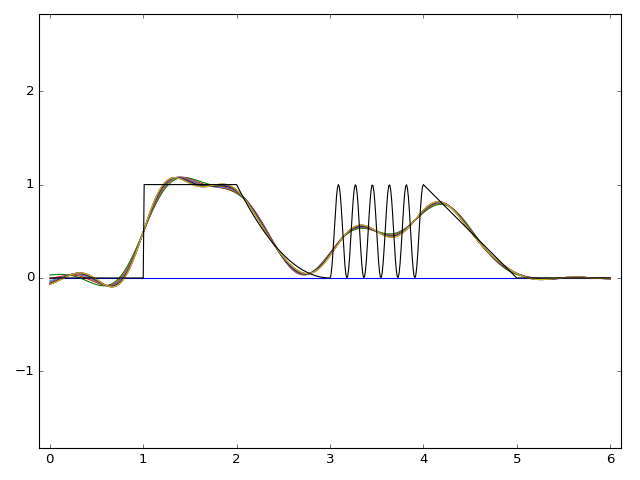Obviously, something did not go as expected!

Computing the $L^2$ gradient. To understand the "smoothing" behavior of the $L^2$ gradient descent in this particular example, we have to open the black-box and actually compute $\partial_x^{L^2} f_{k,y}(x_0)$ at an arbitrary location $x_0$. By definition, it is the unique vector of $X = \mathbb{R}^N$ such that, for all $\delta x \in X$ and $b \in \mathbb{R}$,

$$\langle ~f_{k,y}(x_0 + \delta x)~,~ b \rangle_{\mathbb{R}~} ~=~ \langle~ f_{k,y}(x_0)~,~ b~ \rangle_{\mathbb{R}} ~+~ \langle ~\delta x~,~ \partial_x f_{k,y}(x_0). b ~\rangle_2 ~+~ o(\|\delta x\|_2).$$

Since we know that

$$f_{k,y}(x_0 + \delta x) ~=~ \langle ~(k\star x_0 - y) \,+\, k\star \delta x ~,~ (k\star x_0 - y) \,+\, k\star \delta x ~\rangle_2,$$

we have

$$\partial_x^{L^2} f_{k,y}(x_0) ~=~ 2 ~ \tilde{k} \star (k\star x_0 - y),$$

were $\tilde{k}$ is the mirrored symmetric of $k$, the unique filter such that "$\tilde{k}\star \cdot\,$" is the $L^2$-adjoint of "$k\star \cdot\,$". If $k$ is a centered Gaussian, one simply has $\tilde{k} = k$.

Poorly conditioned operators. Hence, computing the $L^2$ gradient of $f_{k,y}$ involves a smoothing of the difference vector $k\star x_0 - y$. As this operation all but kills the high frequency components, our gradient descent scheme has no way to generate high frequencies in $x$, nevermind $k\star x$. In practice, using the $L^2$ gradient to minimize $f_{k,y}$ is thus akin to enforcing a soft restriction: that of only looking for vectors $x$ which have "the same high frequency content" as the initial guess $x(\text{it}=0)$.

Now, I won't discuss this topic any further. But please note that if $A : \mathbb{R}^N \rightarrow \mathbb{R}^N$ is an invertible linear matrix of size $N$-by-$N$, then $AA^T$ is a symmetric positive definite matrix which defines a metric on $\mathbb{R}^N$:

$$\forall \, x\in \mathbb{R}^N, ~~ \langle x, x \rangle_{AA^T} ~=~ \langle x, AA^T x \rangle_2.$$

Then, if $f : \mathbb{R}^N \rightarrow \mathbb{R}$ is a differentiable function, the minimization of $f$ with an $AA^T$-gradient is equivalent to the minimization of $f \circ A^{-1}$ with an $L^2$ gradient. Using PyTorch, you can thus use other descent metrics without complications.

## An image classification problem¶

These preliminaries in mind, we can now tackle our first image classification problem with a "neural" network. Unlike what was presented in the previous workshop session, we won't add an explicit regularizer to our cross-entropy loss: we rely entirely on the implicit regularization provided by the $L^2$ descent scheme, and hope for the best.

In :
# Train and visualize the performances of our models
from model_utils import AttrDict, show, generate_image, train, test, evaluate_model, display_classified_images

MU.display_parameters()
# MU.use_cuda = False
# MU.args.batch_size = 1000 # A larger batch_size may help you to train at a faster rate, but consumes memory
# MU.args.epochs = 1 # If you just can't wait...

Optimizations will be made using the following arguments:
{'momentum': 0.5, 'log_interval': 10, 'test_batch_size': 250, 'learning_rate': 0.01, 'batch_size': 250, 'epochs': 10}
Using CUDA ?  Yes
Random seed :  1
Remember that you can change those values dynamically!
(MU.args.batch_size = ...)


Our task: classifying into ten groups the images from the "FashionMNIST" dataset of whom you can get an overview at the following address: https://github.com/zalandoresearch/fashion-mnist

In :
# Load the Fashion_MNIST dataset

DATASET = "./fashion_MNIST/" # Storage location
MU.class_names = ['T-shirt', 'Trouser', 'Pullover', 'Dress', 'Coat',
'Sandal',  'Shirt',   'Sneaker',  'Bag',   'Ankle boot' ]
datasets.MNIST.urls = [
'http://fashion-mnist.s3-website.eu-central-1.amazonaws.com/train-images-idx3-ubyte.gz',
'http://fashion-mnist.s3-website.eu-central-1.amazonaws.com/train-labels-idx1-ubyte.gz',
'http://fashion-mnist.s3-website.eu-central-1.amazonaws.com/t10k-images-idx3-ubyte.gz',
'http://fashion-mnist.s3-website.eu-central-1.amazonaws.com/t10k-labels-idx1-ubyte.gz'
]
kwargs = {'num_workers': 2, 'pin_memory': True} if MU.use_cuda else {}

transform=transforms.Compose([
transforms.ToTensor(),
#transforms.Normalize((0.1307,), (0.3081,)) # with normalized values
]))
MU.test_dataset  = datasets.MNIST(DATASET, train=False, transform=transforms.Compose([
transforms.ToTensor(),
#transforms.Normalize((0.1307,), (0.3081,))
]))
# Iterator for the training pass:
batch_size=MU.args.batch_size, shuffle=True, # Data is drawn as randomized minibatches

# Iterator for the testing pass, with the same settings:
batch_size=MU.args.test_batch_size, shuffle=True,
**kwargs)


### Tackling the problem using a multilayer perceptron¶

Just like in the previous workshop session, we can try to "learn" a regression rule by optimizing the weights in a fully connected network. Formally, we're trying to optimize the weights $W_1$, $b_1$ and $W_2$, $b_2$ of a couple of operators $(F_1,F_2)$ given by

$$F_1(x) = \text{Relu}(W_1 \cdot x + b_1)$$$$F_2(x) = \text{argmax} (W_2 \cdot x + b_2)$$

where $\text{Relu}:x \mapsto \max(0, x)$ is applied pointwise, and $\text{argmax}$ goes from $\mathbb{R}^{10}$ to $\{1,2,\cdots,10\}$. The global model $F = F_2\circ F_1$ is the concatenation of those two operators, and we wish to find $F$ such that $F(x_i)$ is as often as possible equal to the label $y_i$ on the test set. That is, we wish to minimize

$$\sum_i 1_{F(x_i) \neq y_i}$$

on the test set, having only tuned the parameters on the "training" set. Because the $\text{argmax}$ operator is piecewise constant, its gradient is pretty uninformative and cannot drive an optimization routine. Hence, we replace it with a softmax operator

$$\text{Softmax}:x \in \mathbb{R}^{10} \mapsto \bigg(\frac{e^{x_i}}{\sum_j e^{x_j}} \bigg)_i \in \mathbb{R}^{10}$$

and strive to minimize the cross-entropy loss seen in the previous workshop session (Softmax Logistic regression).

In :
class TwoFullNet(nn.Module) :
"""
Implements a simplistic perceptron with 3 units :
- one input, of size 28x28 (MNIST dataset)
- one hidden, of size H
- one output, of size 10 (number of classes)
There is no explicit regularization, and we model the two
transformations input->hidden and hidden->output as
Linear+ReLu and Linear+SoftMax operators,
i.e. as Fully connected computational graphs.
The trainable parameters are the weights of the matrices
(+ biases) involved in the "Linear" (Affine, really) operators.
"""
def __init__(self, H) :
"Defines the parameters of the model."
super(TwoFullNet, self).__init__()
# Linear (i.e. fully connected) layer, a matrix of size (28*28)xH
self.fc1        = nn.Linear(MU.imgsize*MU.imgsize, H)
# Linear (i.e. fully connected) layer, a matrix of size Hx10 (10 classes as output)
self.fc2        = nn.Linear( H, 10)

def forward(self, x) :
"""
Apply the model to some input data x.
You can think of x as an image of size 28x28, but it is
actually an Mx28x28 tensor, where M is the size of the
mini-batch.
"""
x = x.view(-1, MU.imgsize*MU.imgsize) # Turns our image into a vector
x = self.fc1( x )     # Linear transformation
x = F.relu(   x )     # Non-linearity (Relu = "positive part", a typical choice)
x = self.fc2( x )     # Second linear transformation
# Really, the softmax is the classification label, but for numerical stability,
# all computations are made in the log domain
return F.log_softmax(x)

two_fc_classifier = TwoFullNet(100)
if MU.use_cuda : two_fc_classifier.cuda()

In :
evaluate_model(two_fc_classifier)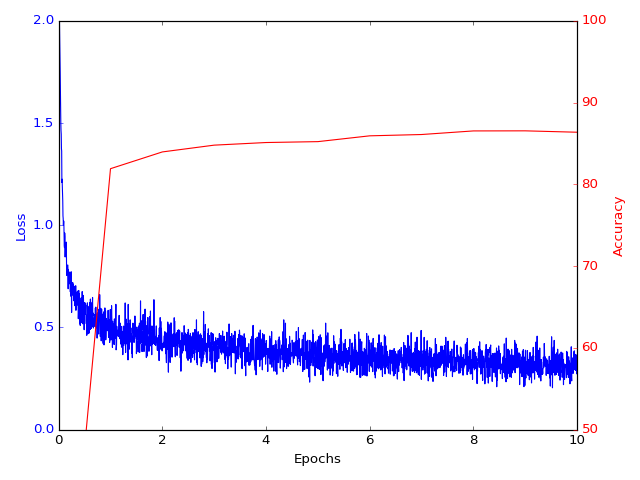Time Elapsed:    1.59s, Average test loss: 2.2900, Test accuracy: 1361/10000 (14%)
Time Elapsed:   71.46s, Average test loss: 0.5164, Test accuracy: 8192/10000 (82%)
Time Elapsed:  142.65s, Average test loss: 0.4605, Test accuracy: 8397/10000 (84%)
Time Elapsed:  206.36s, Average test loss: 0.4330, Test accuracy: 8480/10000 (85%)
Time Elapsed:  285.69s, Average test loss: 0.4207, Test accuracy: 8512/10000 (85%)
Time Elapsed:  357.85s, Average test loss: 0.4128, Test accuracy: 8523/10000 (85%)
Time Elapsed:  434.73s, Average test loss: 0.3936, Test accuracy: 8594/10000 (86%)
Time Elapsed:  502.88s, Average test loss: 0.3922, Test accuracy: 8610/10000 (86%)
Time Elapsed:  573.15s, Average test loss: 0.3774, Test accuracy: 8654/10000 (87%)
Time Elapsed:  650.31s, Average test loss: 0.3744, Test accuracy: 8655/10000 (87%)
Time Elapsed:  719.10s, Average test loss: 0.3830, Test accuracy: 8638/10000 (86%)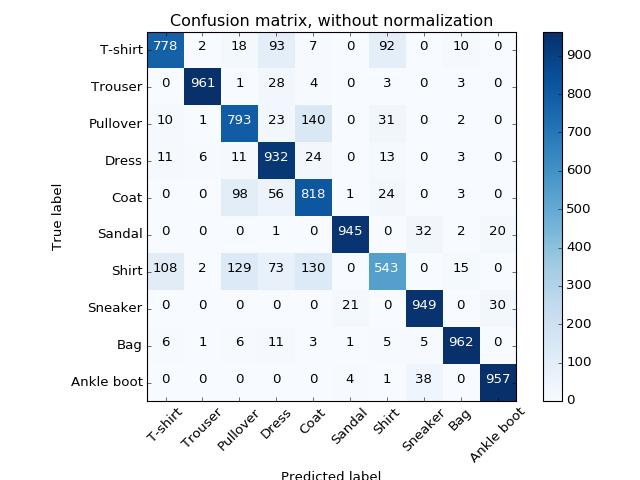Confusion matrix, without normalization


The results are pretty good: most of the confusion comes from classes which are "close" to each other such as "Shirts" and "T-shirts". Even a human operator could get somewhat confused isn't it?

In :
# T-shirs misclassified as shirts
display_classified_images(two_fc_classifier, 0, 6)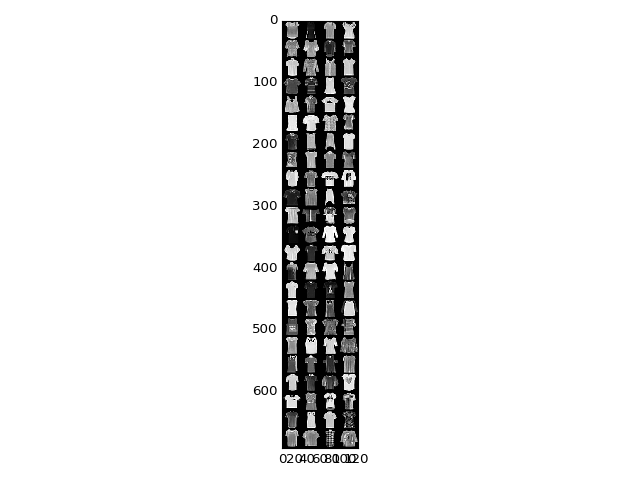To understand the decision rule, we generate (starting from the smooth "null" image, as we want our eye to discern a pattern), by gradient ascent, an image that our network classifies as "100% a T-shirt".

In :
synt_img = generate_image(two_fc_classifier, mode = "class", target_class = 0, seed = 'zero')

fig = plt.figure() ; plt.imshow(synt_img, interpolation='nearest', cmap='gray') ;
plt.tight_layout() ; fig.canvas.draw()

Loss -0.00
Probas per class :  100%, 0%, 0%, 0%, 0%, 0%, 0%, 0%, 0%, 0%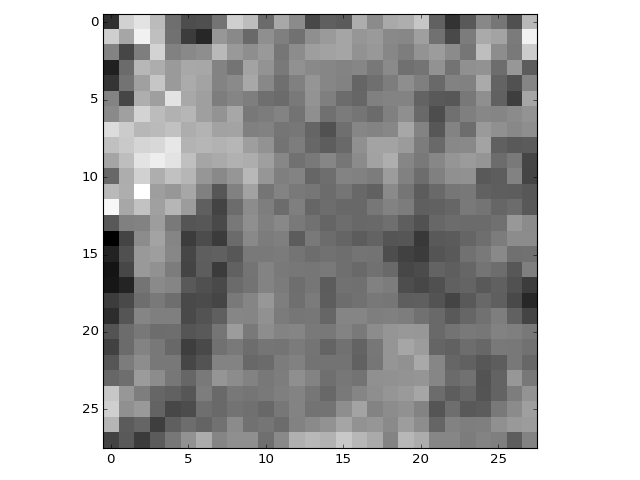We can more or less see the shape of a shirt in there... Try it with other pieces of clothing! Keep in mind tough that our model built a partition of the space of images... without any prior knowledge as to what a natural image should look like! To our model, the image above is every bit as much of a shirt as the one displayed below, generated from a white Gaussian noise.

In :
synt_img = generate_image(two_fc_classifier, mode = "class", target_class = 0, seed = 'random', optim_scheme="Adam")

fig = plt.figure() ; plt.imshow(synt_img, interpolation='nearest', cmap='gray') ;
plt.tight_layout() ; fig.canvas.draw()

Loss 0.00
Probas per class :  100%, 0%, 0%, 0%, 0%, 0%, 0%, 0%, 0%, 0%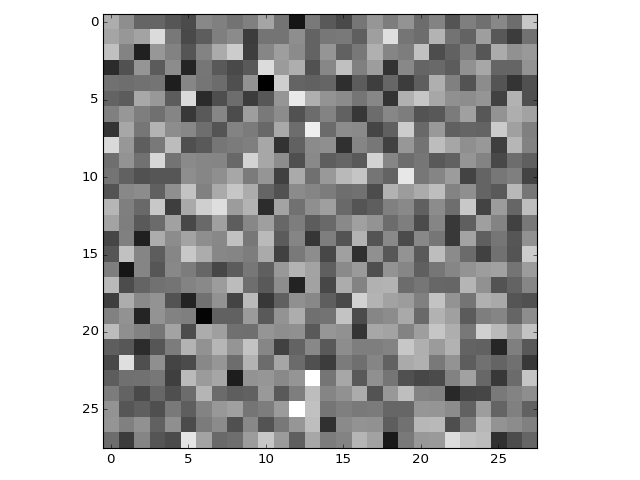You can also investigate the behavior of the first layer using the following syntax.

(N.B.: Since this first layer is linear, you could actually print it directly... but this generic routine should be good enough.)

In :
synt_img = generate_image(two_fc_classifier, mode = "neuron",
target_operator = two_fc_classifier.fc1, target_neuron = (1,),
seed = 'zero')

fig = plt.figure() ; plt.imshow(synt_img, interpolation='nearest', cmap='gray') ;
plt.tight_layout() ; fig.canvas.draw()

Loss -16870.27
Probas per class :  0%, 0%, 0%, 0%, 0%, 0%, 100%, 0%, 0%, 0%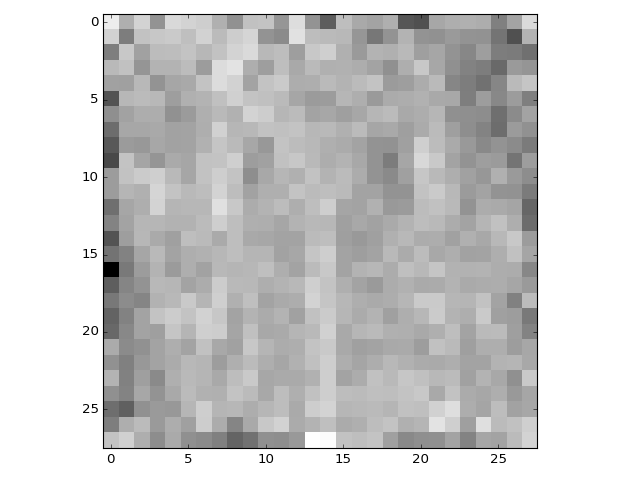## A more sophisticated architecture¶

To gain a few percents, one can finely tune the data flow and create more and more complicated models. Below, we present a model (freely available on GitHub) that was written with this dataset in mind.

Thanks to the flexibility of Autodiff libraries, we can now implement and optimize any model that fits in memory. Given this astounding freedom, the real question thus becomes: how should we design our image processing programs? We will start to answer to this question in the part 2 of the workshop session. For now, let's just execute the code!

In :
class FashionSimpleNet(nn.Module) :
"""
From https://github.com/kefth/fashion-mnist/blob/master/model.py,
originally written by kefth in TensorFlow.
"""
def __init__(self, N) :
"""
Defines the parameters of the model.
The syntax will be explained in Part 2: for now, let's
simply enjoy the fact that we can optimize the parameters
of (pretty much) any "feed-forward" model (i.e. without any
retro-active feedback loop).
"""
super(FashionSimpleNet, self).__init__()
self.conv1 = nn.Conv2d(1,  32, kernel_size=3, padding=1) # 28
self.pool1 = nn.MaxPool2d( 2,  stride     =2)            # 14
self.conv2 = nn.Conv2d(32, 64, kernel_size=3, padding=1) # 14
self.pool2 = nn.MaxPool2d( 2,  stride     =2)            # 7
self.fc1   = nn.Linear(64*7*7, 128)
self.fc2   = nn.Linear(128,    10)
def forward(self, x) :
"""
Apply the model to some input data x.
You can think of x as an image of size 28x28, but it is
actually an Mx28x28 tensor, where M is the size of the
mini-batch.
"""
x = self.pool1(F.relu(self.conv1(x)))
x = self.pool2(F.relu(self.conv2(x)))
x = x.view(-1, 64*7*7)
x = F.dropout(x, training=self.training)
x = self.fc1( x )     # Linear transformation
x = F.relu(   x )     # Non-linearity (Relu = "positive part", a typical choice)
x = self.fc2( x )     # Second linear transformation
# Really, the softmax is the classification label, but for numerical stability,
# all computations are made in the log domain
return F.log_softmax(x)

fashionsimple_classifier = FashionSimpleNet(100)
if MU.use_cuda : fashionsimple_classifier.cuda()

In :
evaluate_model(fashionsimple_classifier)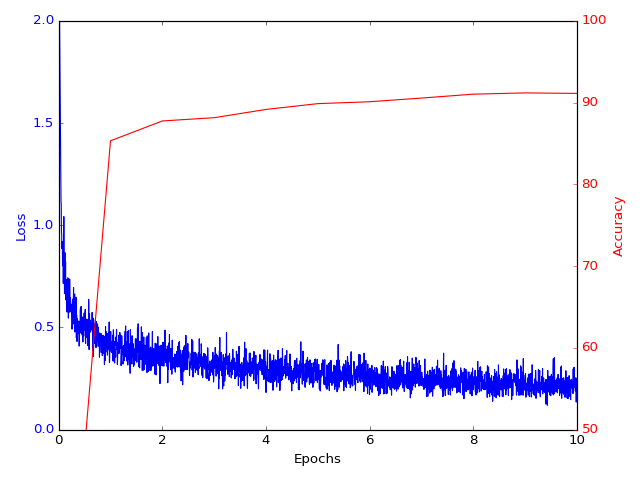Time Elapsed:    2.48s, Average test loss: 2.3016, Test accuracy: 1001/10000 (10%)
Time Elapsed:   68.12s, Average test loss: 0.4125, Test accuracy: 8533/10000 (85%)
Time Elapsed:  134.82s, Average test loss: 0.3439, Test accuracy: 8776/10000 (88%)
Time Elapsed:  207.04s, Average test loss: 0.3202, Test accuracy: 8816/10000 (88%)
Time Elapsed:  280.33s, Average test loss: 0.2941, Test accuracy: 8917/10000 (89%)
Time Elapsed:  352.07s, Average test loss: 0.2739, Test accuracy: 8988/10000 (90%)
Time Elapsed:  426.03s, Average test loss: 0.2698, Test accuracy: 9011/10000 (90%)
Time Elapsed:  502.95s, Average test loss: 0.2580, Test accuracy: 9056/10000 (91%)
Time Elapsed:  574.33s, Average test loss: 0.2441, Test accuracy: 9104/10000 (91%)
Time Elapsed:  647.43s, Average test loss: 0.2401, Test accuracy: 9119/10000 (91%)
Time Elapsed:  717.49s, Average test loss: 0.2412, Test accuracy: 9113/10000 (91%)